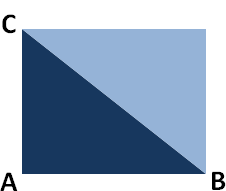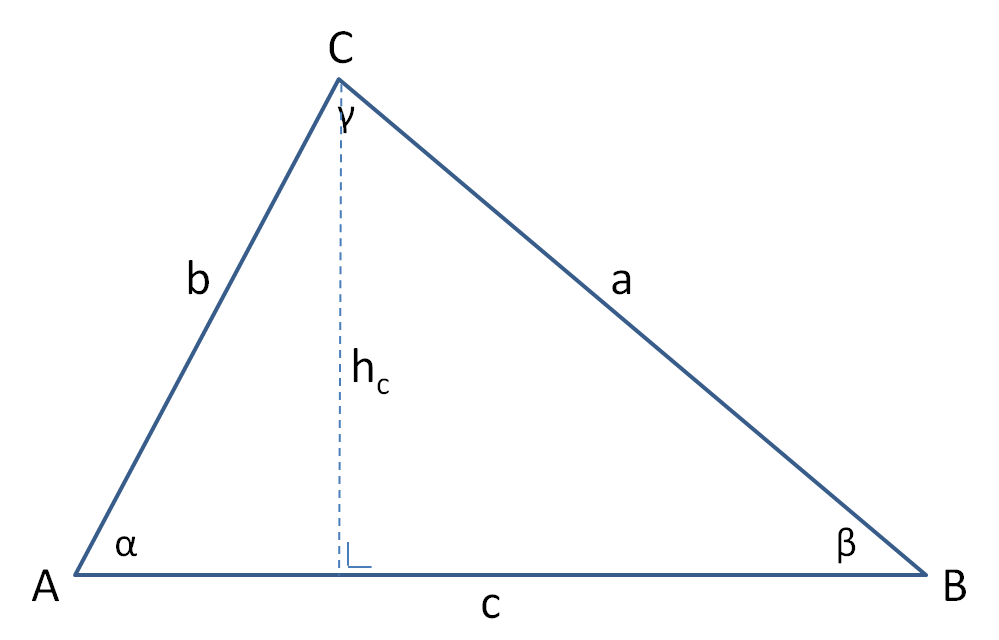# Area of a triangle

The area of a triangle can be calculated by multiplying the base by the height, and then divide the result by 2. In order to find the height and area of the triangle, you can fit the triangle in a rectangle. The area of the triangle is half the area of this rectangle.

### Area triangle = (BASE x HEIGHT)/2

When we have a right-angled triangle (see the left image below), you can determine the heigth quite easy: if one leg is taken as the base (line A-B) then the other is height (line A-C).

When we have a triangle without a right angle (see the right image below), it is less easier to determine the area of the triangle. However if we draw a line from the angle C perpendicular to the base (the line between A and B) we can measure the altitude or height of our triangle. Now we can calculate the area again by multiplying the base by the height, divided by 2.Right-angled triangle: the height is the side perpendicular to the base.Triangle without a right angle: the height is the line from the angle C perpendicular to the base.

If some values (sides and/or angles) of the triangle are known, it is possible to calculate the height of the triangle from a certain angle. Depending on which information is given, you can determine the altitude of the triangle from one of the three angles. Each angle has its own altitude perpendicular to the opposite side (the base), see the left image below. In order to calculate the height you can use the triangle formulas below. After you have calculate the height, you can calculate the area of te triangle by multiplying the base by the height, divided by 2.Triangle altitude from each angle.

### ha = b * sin(angle C) orha = c * sin(angle B)

If at least 3 values are known 3 including at least one side, you can use triangle formulas or the triangle calculator below to calculate the area of a triangle.

## Calculate the area of a triangle

Enter 3 values including at least one side. The triangle values will be calculated based on 3 input values. When more values are entered, they will be overwritten. A graphic representation of the triangle will be presented after the calculation has been made.

#### Triangle calculator

 Side a: Side b: Side c (base): Angle A (α): Angle B (β): Angle C (γ): Height (hc): Area: Perimeter : Enter 3 values, of which at least one side.

#### Triangle ABC (example)